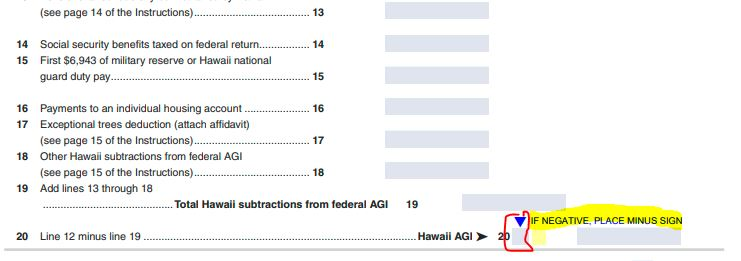cancel
Showing results for
Did you mean:Level 1

# Arithmetic error in Hawaii state return N-11 2020

It looks like Turbotax made arithmetic errors on my Hawaii state return.

1. Line 20 of N-11 2020, which is the Hawaii AGI, is supposed to be the amount of Line 12 minus Line 19.  My Line 19 is larger than Line 12, but the amount on Line 20 is positive instead of negative.  It is the same amount as Line 19 minus Line 12 (the inverse of the operation which the tax return describes.)  Shouldn't the minus sign appear if it is a negative number?  I do see in the Itemized Deductions Worksheet that it is shown as a negative on line 2.

2. Similarly, Line 24 is supposed to be Line 20 minus Line 22 or 23 (whichever applies.)  Turbotax filled in Line 22, which is larger than Line 20.  But Line 24 shows the SUM of Line 20 and 22, not the difference between them, which would be negative.

3. On the N-11 Itemized Deductions Worksheet, Gifts to Charity are entered correctly on lines 15 and 16.  However, Line 18, which is supposed to be the sum of lines 15 through 17, is shown as 0.  This amount (0) is shown on Form N-11, line 21d as well, and thus not reflected in Form N-11 Line 22.

Is there a glitch in the software, or am I missing something???

2 RepliesEmployee Tax Expert

## Arithmetic error in Hawaii state return N-11 2020

If you look on Line 20 on the actual form, that number is always positive, but there is a box to the left of it where you would enter a "-" sign to indicate that the number is negative. If line 20 was negative, then line 23 should be added to it to arrive at line 24, since they are both subtractions.It may be that you have the standard deduction of \$2,200 (single) or \$4,400 (joint filer) in box 23, so the charitable contributions are not tax deductible.

**Say "Thanks" by clicking the thumb icon in a postLevel 1

## Arithmetic error in Hawaii state return N-11 2020

Thanks for illustrating where the negative sign is supposed to go on line 20.  I see an "x" in that space, instead of a "-" so I will have to hope that the "x" also means a negative.   I also see an "x" next to line 24, so I'm going to assume that TurboTax is adding two negatives, as you said.

Perhaps I am taking the standard deduction, though it is not filled in on line 23.  I assumed that it wasn't filled in because line 24 shows "Line 20 minus line 22 or 23, whichever applies. (This line MUST be filled in)."  Line 24 has that negative number, line 20 minus my total itemized deductions.

Thanks for your insights.  If I have more questions, I'll post them!

v
Privacy Settings